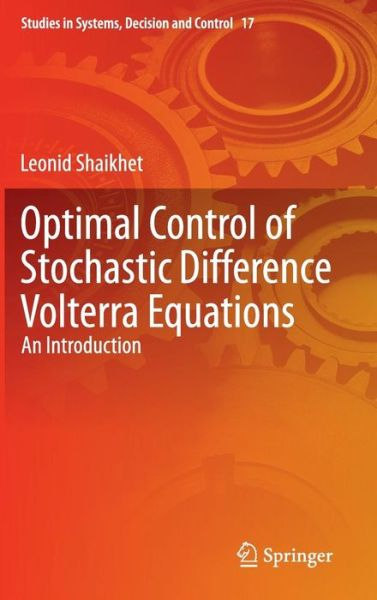## Leonid Shaikhet

Pris
NOK 1.349
Forventes levert 22. jun - 3. jul

# Optimal Control of Stochastic Difference Volterra Equations: An Introduction - Studies in Systems, Decision and Control 2015 edition

This book showcases a subclass of hereditary systems, that is, systems with behaviour depending not only on their current state but also on their past history; it is an introduction to the mathematical theory of optimal control for stochastic difference Volterra equations of neutral type. As such, it will be of much interest to researchers interested in modelling processes in physics, mechanics, automatic regulation, economics and finance, biology, sociology and medicine for all of which such equations are very popular tools.

The text deals with problems of optimal control such as meeting given performance criteria, and stabilization, extending them to neutral stochastic difference Volterra equations. In particular, it contrasts the difference analogues of solutions to optimal control and optimal estimation problems for stochastic integral Volterra equations with optimal solutions for corresponding problems in stochastic difference Volterra equations.

Optimal Control of Stochastic Difference Volterra Equations commences with an historical introduction to the emergence of this type of equation with some additional mathematical preliminaries. It then deals with the necessary conditions for optimality in the control of the equations and constructs a feedback control scheme. The approximation of stochastic quasilinear Volterra equations with quadratic performance functionals is then considered. Optimal stabilization is discussed and the filtering problem formulated. Finally, two methods of solving the optimal control problem for partly observable linear stochastic processes, also with quadratic performance functionals, are developed.

Integrating the author?s own research within the context of the current state-of-the-art of research in difference equations, hereditary systems theory and optimal control, this book is addressed to specialists in mathematical optimal control theory and to graduate students in pure and applied mathematics and control engineering.

220 pages, 8 colour illustrations, biography

 Media Bøker     Innbunden bok   (Bok med hard rygg og stivt omslag) Utgitt Schweiz, 9. desember 2014 ISBN13 9783319132389 Utgivere Springer International Publishing AG Antall sider 220 Mål 155 × 235 × 14 mm   ·   503 g Språk Engelsk

Vis alle